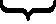Study of mathematics online.
Study math with us and make sure that "Mathematics is easy!"

# Formulas and properties of exponents

Definition
When n is a positive integer, the number c is called the n power of the number a if
 c = an = a · a · ... · an

Formulas and properties of exponents are used in the reduction and simplification of expressions, and in solving equations and inequalities.

 1 a0 = 1      (a ≠ 0) - zero exponent property 2 a1 = a - any number raised by the exponent 1 is the number itself 3 an · am = an + m - product of powers property 4 (an)m = anm - power of a power property 5 anbn = (ab)n - power of a product property 6 a-n = 1an - negative exponent property 7 anam = an - m - quotient of powers property 8 a1/n = n√a - rational exponent property